# Folding a square into thirds

Alignments to Content Standards: G-SRT.B.5

Suppose we take a square piece of paper and fold it in half vertically and diagonally, leaving the creases shown below: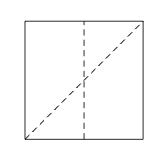Next a fold is made joining the top of the vertical crease to the bottom right corner, leaving the crease shown below: the point $P$ is the intersection of this new crease with the diagonal.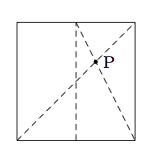In the diagram below, some additional points are labelled: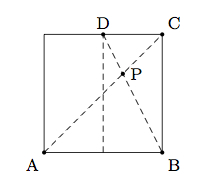1. Show that $|AP| = 2|CP|$.
2. Using part (a), explain how to use the point $P$ in order to fold the square into equal thirds.

## IM Commentary

The goal of this task is to apply knowledge about similar triangles in order to understand an origami construction: trisection of the side of a square. The task stem provides the basic method of construction and then students will need to explain how it works. There are many other closely related approaches based upon similarity of different pairs of triangles: for example, in the picture below $\triangle DPE$ is similar to $\triangle CPB$Or, if $H$ is the intersection of $\overleftrightarrow{PD}$ with $\overleftrightarrow{AF}$, then $\triangle AHP$ is similar to $\triangle CBP$. In order to give a flavor of the different similarity arguments available, we present an alternate proof of part (b) using a horizontal line through $P$ (shown in purple in the picture above) instead of a vertical line.

An algebraic version of this task, using coordinates, is given in www.illustrativemathematics.org/illustrations/1571. Teachers may wish to compare and contrast the solution given there for part (b) which is briefly mentioned here without details.

One practice standard closely related to this task is MP7, Look For and Make Use of Structure. Students will need to idenitfy, amongst the many triangles made by the paper folds, similar triangles and then use these to set up the appropriate proprotions which reveal the location of the point $P$.

Here is an image of a modified omega origami star that begins by folding the paper into thirds:## Solutions

Solution: 1

1. To get our ratio between side lengths we will study $\triangle APB$ and $\triangle CPD$, showing that they are similar with a scale factor of $\frac{1}{2}$ to go from $\triangle APB$ to $\triangle CPD$. First, to see that they are similar, note that <\p>

• $m(\angle BAP) = (\angle PCD)$ as these are alternate interior angles formed by the transverse $\overleftrightarrow{AC}$ meeting opposite (parallel) sides of the square.
• $m(\angle PBA) = (\angle PDC)$ as these are alternate interior angles formed by the transverse $\overleftrightarrow{DB}$ meeting opposite (parallel) sides of the square.

By the AA triangle similarity criterion, it follows that $\triangle APB$ and $\triangle CPD$.

To find the scale factor between $\triangle APB$ and $\triangle CPD$, we know that $|AB| = 2|CD|$ because $D$ is on the fold bisecting opposite sides of the square. Since $\overline{AB}$ and $\overline{CD}$ are corresponding parts of similar triangles, this means that the scale factor to go from $\triangle APB$ to $\triangle CPD$ is $\frac{1}{2}$. Since $\overline{AP}$ and $\overline{CP}$ are corresponding parts of the similar triangles $\triangle APB$ and $\triangle CPD$ we can conclude that $|CP| = \frac{1}{2}|AP|$ or $$|AP| = 2|CP|$$ as desired.

2. Intuitively, we know from the first part of the problem that $P$ is two thirds of the way from $A$ to $B$. This means that it is two thirds of the way from the lower edge to the upper edge of the square and also two thirds of the way from the left edge to the right edge of the square. Hence folding the left edge of the square over to $P$ will produce a vertical crease $\frac{1}{3}$ of the width of the square.

To make this argument rigorous, we could use an explicit coordinate system. Instead, to reinforce the similarity argument of part (a), we add a vertical line segment through $P$: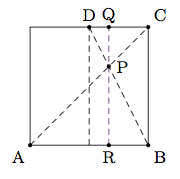We know that $\overline{PQ}$ is an altitude of $\triangle CPD$. We also know that $\overline{PR}$ is the corresponding altitude of $\triangle APB$. Since the two triangles are similar with scale factor $\frac{1}{2}$ going from $\triangle APB$ to $\triangle CPD$, this means that $|PQ| = \frac{1}{2}|PR|$ of $|PR| = 2|PQ|$. Since $|BC| = |PQ| + |PR|$, we see that $|BC| = 3|PQ|$. Thus to fold the square in equal thirds horizontally, we can fold side $\overline{AB}$ up to $P$ (making a crease one third of the way from $B$ to $C$) and then fold the opposite side down to the new crease (making a crease two thirds of the way from $B$ to $C$).

Solution: 2 Alternate solution for part (b)

We can add a horizontal line, through $P$, perpendicular to $\overleftrightarrow{BC}$, and make another use of similar triangles.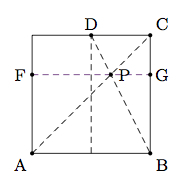We claim that $\triangle FPA$ is similar to $\triangle GPC$ with scale factor $\frac{1}{2}$. We have

• $m(\angle PFA) = (\angle PGC)$ since these are both right angles: this is true of $\angle PGC$ by construction and $\overleftrightarrow{AF}$ is parallel to $\overleftrightarrow{BC}$ so $\overleftrightarrow{FG}$ is also perpendicular to $\overleftrightarrow{AF}$.
• $m(\angle FPA) = (\angle GPC)$ since these are vertical angles.

By the AA criterion for similarity, $\triangle FPA$ is similar to $\triangle GPC$. The scale factor is $$\frac{|CP|}{|AP|} = \frac{1}{2}$$ from part (a). Hence $|GC| = \frac{1}{2}|FA|$ or, equivalently $|FA| = 2|GC|$. Since $|GB| = |FA|$ we get

\begin{align} |BC| &= |GC| + |GB| \\ &= |GC| + 2|GC| \\ &= 3|GC|. \end{align}

This means that the crease $\overline{FG}$ trisects the vertical sides of the square. In order to get the other vertical division, $\frac{2}{3}$ of the way from $C$ to $B$, we can fold $\overline{AB}$ up to $\overline{FG}$ and the new crease will be $\frac{2}{3}$ of the way from $C$ to $B$.